# Let Me Graph That For You – Part 1 – Air Routes

We’re pleased to announce the start of a multi-part series of posts for Amazon Neptune in which we explore graph application datasets and queries drawn from many different domains and problem spaces.

Amazon Neptune is a fast and reliable, fully-managed graph database, optimized for storing and querying highly connected data. It is ideal for online applications with highly connected data where the query workloads require you to take advantage of this connectedness – navigating connections and leveraging the strength, weight or quality of the relationships between entities. If you’ve ever had to answer questions such as:

• Which friends and colleagues do we have in common?
• Which applications and services in my network will be affected if a particular network element – a router or switch, for example – fails? Do we have redundancy throughout the network for our most important customers?
• What’s the quickest route between two stations on the underground?
• What do you recommend this customer should buy, view, or listen to next?
• Which products, services and subscriptions does a user have permission to access and modify?
• What’s the cheapest or fastest means of delivering this parcel from A to B?
• Which parties are likely working together to defraud their bank or insurer?

– then you’ve already encountered the need to manage and make sense of highly connected data.

We kick off our series with an open source air routes dataset that models the world airline route network. This dataset accompanies the book Practical Gremlin.

All the examples in this series will be presented as Jupyter notebooks using the Amazon SageMaker and Neptune integration solution described in Analyze Amazon Neptune Graphs using Amazon SageMaker Jupyter Notebooks. Each notebook contains sample data and queries, together with commentary on the data model and query design techniques used to address the application use case.

### Launch the Air Routes dataset

To launch the Neptune-SageMaker stack from the AWS CloudFormation console, choose one of the Launch Stack buttons in the following table. Select the check box to acknowledge that AWS CloudFormation will create IAM resources. Then choose Create.

Note: The Neptune and SageMaker resources deployed in this solution incur costs. With SageMaker hosted notebooks you pay simply for the EC2 instance hosting the notebook. In this blog post, we use an ml.t2.medium instance, which is eligible for the AWS Free Tier.

 Region View Launch US East 1 (N. Virginia) View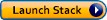US East 2 (Ohio) ViewUS West 2 (Oregon) ViewEU West 1 (Ireland) ViewEU West 2 (London) ViewEU Central 1 (Frankfurt) ViewOnce the stack has been created, open the Amazon SageMaker console and from the left-hand menu select Notebook instances. Click the Open link next to the Neptune notebook instance.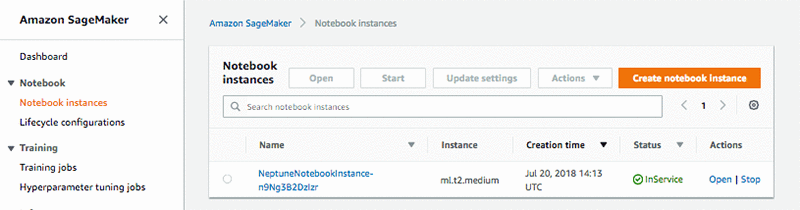In the Jupyter window, open the Neptune directory, and then the Let-Me-Graph-That-For-You subdirectory. Then open the 01-Air-Routes.ipynb notebook.

## The Air Routes Dataset

The air routes dataset models a large part of the world airline route network. It contains vertices representing 3,397 airports, 237 countries and provinces, and the 7 continents. Edges are used to represent the routes between the airports and the links between continents, countries and airports. There are 52,639 edges in the graph, of which 45,845 represent airline routes.

The air routes data set is available in GraphML format, but Neptune’s bulk loader API expects CSV formatted files. To convert GraphML to CSV we used graphml2csv.py, which is available from the AWS Labs GitHub project. We then put copies of the resulting files in region-specific S3 buckets. Your notebook will load data into Neptune from an S3 bucket located in the same region as your Neptune and SageMaker instances.

Our notebook shows how to use gremlinpython to connect to and work with a Neptune instance.

The notebook uses a helper model, neptune.py, to load data into the graph using Neptune’s loader API. This helper code then establishes a connection to Neptune and binds the variable g to a graph traversal source. We use g for all subsequent gremlinpython queries. (You can read more about neptune.py in the post describing our Neptune-SageMaker solution.)

### Let’s find out a bit about the graph

We start with a simple query just to make sure our connection to Neptune is working. The queries below look at all of the vertices and edges in the graph and create two maps that show the demographic of the graph. As we are using the air routes data set, not surprisingly the values returned are related to airports and routes.

``````vertices = g.V().groupCount().by(T.label).toList()
edges  = g.E().groupCount().by(T.label).toList()
print(vertices)
print(edges)``````

When you execute these queries in the notebook, you’ll see the following results:

``````[{'continent': 7, 'country': 237, 'version': 1, 'airport': 3397}]
[{'contains': 6794, 'route': 45845}]``````

### Find routes longer than 8,400 miles

In the next query we find routes in the graph that are longer than 8,400 miles. This is done by examining the `dist` property of the `routes` edges in the graph. Having found some edges that meet our criteria we sort them in descending order by distance. The `where` step filters out the reverse direction routes for the ones that we have already found because we only want one result for each route. Finally, we generate some `path` results using the airport codes and route distances.

(Notice how in the notebook code we have laid the Gremlin query out over multiple lines to make it easier to read. To avoid errors, when you lay out a query in this way using Python, each line must end with a backslash character, “\”.)

The results from running the query will be placed into the variable `paths`. Notice how we ended the Gremlin query with a call to `toList()`. This tells Gremlin that we want our results back in a list. We can then use a Python `for` loop to `print` those results. Each entry in the list will itself be a list containing the starting airport code, the length of the route and the destination airport code.

Here’s the query:

``````paths =  g.V().hasLabel('airport').as_('a') \
.outE('route').has('dist',gt(8400)) \
.order().by('dist',Order.decr) \
.inV() \
.where(P.lt('a')).by('code') \
.path().by('code').by('dist').by('code') \
.toList()

for p in paths:
print(p)
``````

Executing the code in the notebook generates the following results:

``````['DOH', 9025, 'AKL']
['PER', 9009, 'LHR']
['PTY', 8884, 'PEK']
['DXB', 8818, 'AKL']
['SIN', 8756, 'LAX']
['MEX', 8754, 'CAN']
['SYD', 8591, 'IAH']
['SYD', 8574, 'DFW']
['JNB', 8434, 'ATL']
['SIN', 8433, 'SFO']``````

### Draw a Bar Chart that represents the routes we just found

One of the nice things about using Python to work with our graph is that we can take advantage of the larger Python ecosystem of libraries such as matplotlib, numpy and pandas to further analyze our data and represent it pictorially. So, now that we have found some long airline routes we can build a bar chart that represents them graphically:

``````import matplotlib.pyplot as plt; plt.rcdefaults()
import numpy as np
import matplotlib.pyplot as plt
import pandas as pd

routes = list()
dist = list()

# Construct the x-axis labels by combining the airport pairs we found
# into strings with with a "-" between them. We also build a list containing
# the distance values that will be used to construct and label the bars.
for i in range(len(paths)):
routes.append(paths[i] + '-' + paths[i])
dist.append(paths[i])

# Setup everything we need to draw the chart
y_pos = np.arange(len(routes))
y_labels = (0,1000,2000,3000,4000,5000,6000,7000,8000,9000)
freq_series = pd.Series(dist)
plt.figure(figsize=(11,6))
fs = freq_series.plot(kind='bar')
fs.set_xticks(y_pos, routes)
fs.set_ylabel('Miles')
fs.set_title('Longest routes')
fs.set_yticklabels(y_labels)
fs.set_xticklabels(routes)
fs.yaxis.set_ticks(np.arange(0, 10000, 1000))
fs.yaxis.set_ticklabels(y_labels)

# Annotate each bar with the distance value
for i in range(len(paths)):
fs.annotate(dist[i],xy=(i,dist[i]+60),xycoords='data',ha='center')

# We are finally ready to draw the bar chart
plt.show()``````

Running this code in the notebook generates the following chart: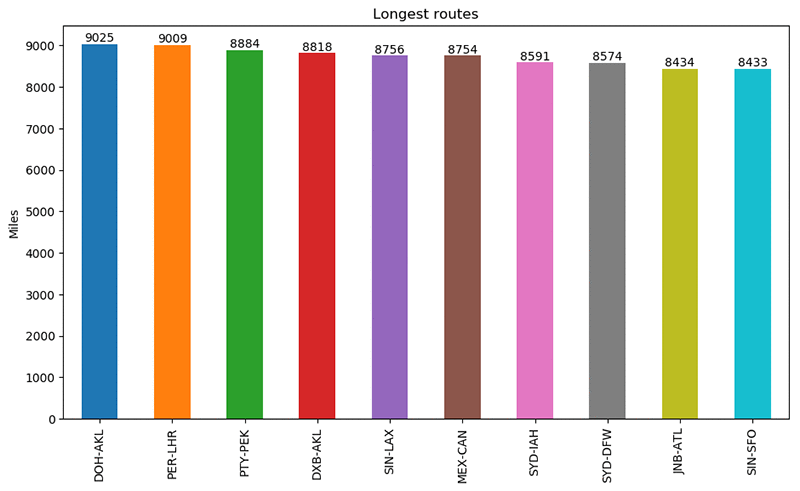#### Explore the distribution of airports by continent

The next example queries the graph to find out how many airports are in each continent. The query starts by finding all vertices that are continents. Next, the query groups the vertices to create a map (or dict) whose keys are the continent descriptions and whose values represent the counts of the outgoing edges with a `contains` label. Finally, the resulting map is sorted using the keys in ascending order. That result is then returned to our Python code as the variable `m`, which we iterate over and print to the output:

``````# Return a map where the keys are the continent names and the values are the
# number of airports in that continent.
m = g.V().hasLabel('continent') \
.group().by('desc').by(__.out('contains').count()) \
.order(Scope.local).by(Column.keys) \
.next()

for c,n in m.items():
print('%4d %s' %(n,c))
``````

Executing the code in the notebook generates the following results:

``````295 Africa
0 Antarctica
939 Asia
596 Europe
980 North America
285 Oceania
305 South America``````

#### Draw a pie chart representing the distribution by continent

Rather than return the results as text like we did above, it might be nicer to display them as percentages on a pie chart. That is what the next piece of code does, using the two-digit character code for each continent to label the chart:

``````import matplotlib.pyplot as plt; plt.rcdefaults()
import numpy as np

# Return a map where the keys are the continent codes and the values are the
# number of airports in that continent.
m = g.V().hasLabel('continent').group().by('code').by(__.out().count()).next()

fig,pie1 = plt.subplots()

pie1.pie(m.values() \
,labels=m.keys() \
,autopct='%1.1f%%'\
,startangle=90 \
,explode=(0,0,0.1,0,0,0,0))

pie1.axis('equal')

plt.show()``````

This code generates the following pie chart: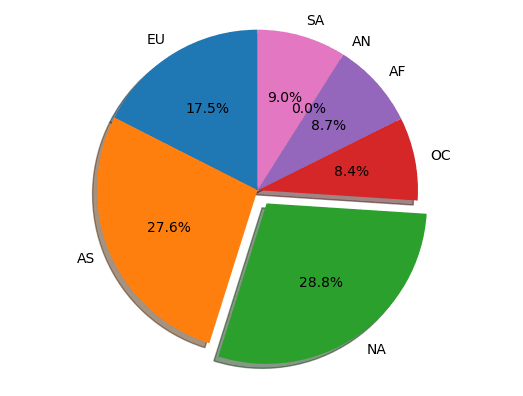### Find some routes from London to San Jose and draw them

One of the nice things about connected graph data is that it lends itself nicely to creating useful visualizations. The Python networkx library makes it fairly easy to draw a graph. The next example in our notebook takes advantage of this capability to draw a directed graph (DiGraph) of a few airline routes.

The query below starts by finding the vertex that represents London’s Heathrow (LHR) airport. It then finds 15 routes from LHR that end up in San Jose California (SJC) with one stop on the way. Those routes are returned as a list of paths. Each path will contain the three-character IATA codes representing the airports found.

The main purpose of this example is to show that we can easily extract part of a larger graph and render it graphically in a way that is easy for an end user to understand.

``````import matplotlib.pyplot as plt; plt.rcdefaults()
import numpy as np
import matplotlib.pyplot as plt
import pandas as pd
import networkx as nx

# Find up to 15 routes from LHR to SJC that make one stop.
paths = g.V().has('airport','code','LHR') \
.out().out().has('code','SJC').limit(15) \
.path().by('code').toList()

# Create a new empty DiGraph
G=nx.DiGraph()

# Add the routes we found to DiGraph we just created
for p in paths:

# Give the starting and ending airports a different color
colors = []

for label in G:
if label in['LHR','SJC']:
colors.append('yellow')
else:
colors.append('#11cc77')

# Now draw the graph
plt.figure(figsize=(5,5))
nx.draw(G, node_color=colors, node_size=1200, with_labels=True)
plt.show()``````

Here’s the graphical output of executing this code in the notebook: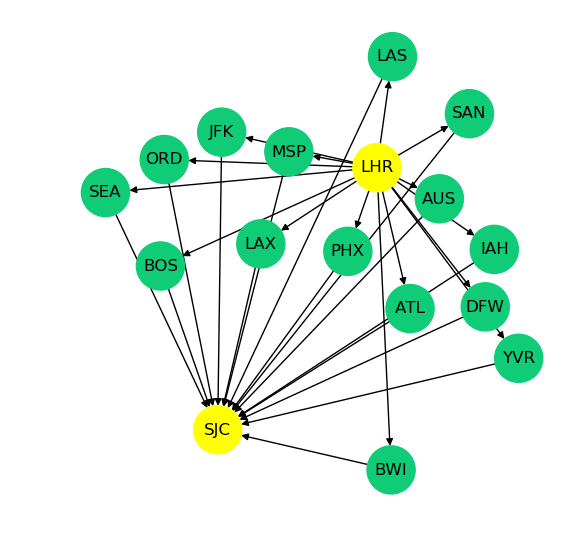## Conclusion

In this post we’ve shown how to load and query a highly connected air routes dataset, and produce bar charts, pie charts and graph diagrams based on the results of those queries.

Look out for subsequent posts in the Let Me Graph That For You series. If you’ve a particular application use case you’d like us to address, let us know in the comments.Kelvin Lawrence is a Principal Data Architect in the Database Services Customer Advisory Team focused on Amazon Neptune and many other related services. He has been working with graph databases for many years, is the author of the book “Practical Gremlin” and is a committer on the Apache TinkerPop project.Ian Robinson is an architect with the Database Services Customer Advisory Team. He is a coauthor of ‘Graph Databases’ and ‘REST in Practice’ (both from O’Reilly) and a contributor to ‘REST: From Research to Practice’ (Springer) and ‘Service Design Patterns’ (Addison-Wesley)

TAGS: